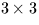Next: SLA_PV2EL - Orbital Elements from Position/Velocity
Up: SUBPROGRAM SPECIFICATIONS
Previous: SLA_PRECL - Precession Matrix (latest)

## SLA_PRENUT - Precession/Nutation Matrix

ACTION:
Form the matrix of precession and nutation (IAU 1976, FK5).
CALL:
CALL sla_PRENUT (EPOCH, DATE, RMATPN)

GIVEN:

 D EPOCH Julian Epoch for mean coordinates DATE D Modified Julian Date (JD-2400000.5) for true coordinates

RETURNED:

 D(3,3) RMATPN combined precession/nutation matrix

NOTES:
1.
The epoch and date are TDB.
2.
The matrix is in the sense:
vtrue = Mvmean
where vtrue is the star vector relative to the true equator and equinox of epoch DATE, M is thematrix RMATPN and vmean is the star vector relative to the mean equator and equinox of epoch EPOCH.Next: SLA_PV2EL - Orbital Elements from Position/Velocity
Up: SUBPROGRAM SPECIFICATIONS
Previous: SLA_PRECL - Precession Matrix (latest)

SLALIB --- Positional Astronomy Library